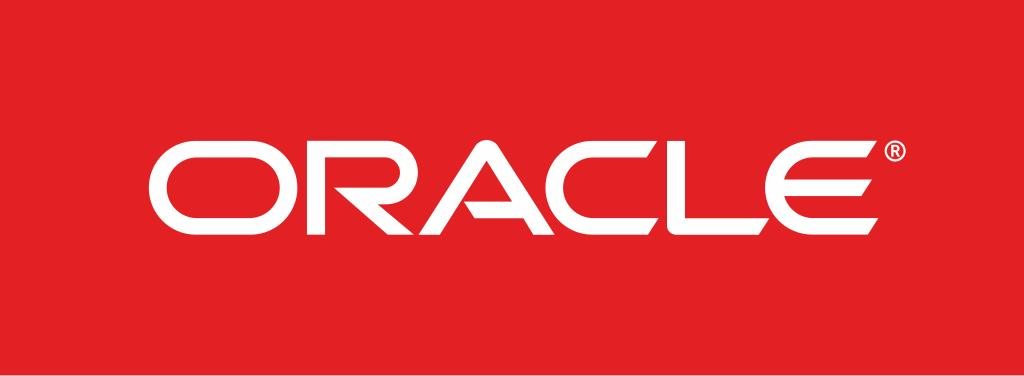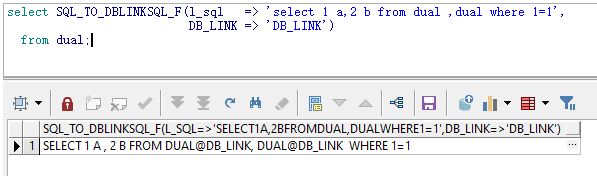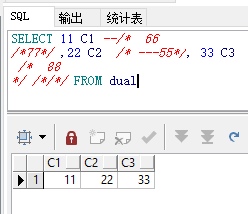### 二、分析

``````select col1,col2 from tmp_table where col1=2
``````

``````select col1,col2 from tmp_table@DBLINK where col1=2
``````

``````select (SELECT EEE FROM TTT WHERE T1=456),* from tab5,
(select * from
taba1 , taba2 b ,TAB3

),(select   user_from  /*这里是注释 FROM TAB */  from tabfrom

) where 1=1
``````

``````select (SELECT EEE FROM TTT@DBLINK WHERE T1=456),* from tab5@DBLINK,
(select * from

),(select   user_from  /*这里是注释 FROM TAB */  from tabfrom@DBLINK

) where 1=1
``````

1.处理掉所有回车让其变成一行，
2.两个空格的都缩成一个空格，
3.再统一大小写
(这些操作可能会导致SQL查询结果发生变化，因此能使用的SQL会受到一些限制,比如这些都是在静态字符串中 类似 select 'AbcD' from tab where id='98 54321' 这样的)

``````SELECT (SELECT EEE FROM TTT WHERE T1=456),* FROM TAB5,(SELECT * FROM TABA1 , TABA2 B ,TAB3),(SELECT USER_FROM /*这里是注释 FROM TAB */ FROM TABFROM ) WHERE 1=1
``````

1.表的前面某个位置一定能找到“FROM”，但“FROM”后面不一定就是表
2.“FROM”可以在表名或者字段名中出现，那么真正意义上的“from”应该前后都有空格，即“ FROM ”
3.表的后面如果有逗号，且逗号后面不是括号，那么逗号后面的一定也是个表
4. "/*" 后面的某个位置如果有 "*/" ，那么这两个中间的就是注释,不管里面有什么都要忽略掉，否则可能影响判断，
5. 表的后面可能有别名
6. " FROM "后面找表，直到“ WHERE ”就停，然后找下一个" FROM "

### 三、成果

``````CREATE OR REPLACE FUNCTION SQL_TO_DBLINKSQL_F(l_sql varchar2,DB_LINK VARCHAR2) RETURN VARCHAR2 IS
/*

you may not use this file except in compliance with the License.
You may obtain a copy of the License at

Unless required by applicable law or agreed to in writing, software
WITHOUT WARRANTIES OR CONDITIONS OF ANY KIND, either express or implied.
See the License for the specific language governing permissions and
*/

2：FROM后面表的顺序必须是先表后子查询
3：查询字段允许使用子查询
4：查询条件中的静态字符串不要有 A.大小写混合，B.大于1个以上的空格，C.回车*/
str   varchar2(30000);
str2  varchar2(30000);
str_a varchar2(30000);
str_b varchar2(30000);
str_T varchar2(30000);

STR2_L NUMBER;
I      NUMBER;
J      NUMBER;
K      NUMBER;

L NUMBER;
begin
l    := 0;
/* str  := 'select (SELECT EEE FROM TTT WHERE T1=456),* from
(select * from
taba1 , taba2 b ,TAB3

),(select   user_from    from tabfrom

) where 1=1
';*/
str  := l_sql;
str2 := UPPER(str); ---大写
str2 := replace(str2, chr(10), chr(32)); ---回车转空格
str2 := replace(str2, chr(9), chr(32)); ---TAB转空格
str2 := replace(str2, chr(44), chr(32) || chr(44) || chr(32)); ---逗号前面后面加空格
str2 := replace(str2, chr(40), chr(32) || chr(40) || chr(32)); ---括号前面后面加空格
str2 := replace(str2, chr(41), chr(32) || chr(41) || chr(32)); ---括号前面后面加空格

--- STR2_L:=LENGTH(STR2);

LOOP
STR2_L := LENGTH(STR2);
STR2   := REPLACE(STR2, CHR(32) || CHR(32), CHR(32)); ---双空格替换成单空格
IF STR2_L = LENGTH(STR2) THEN
EXIT;
END IF;
END LOOP;
I     := 1;
str_b := STR2;
--- str_a:=SUBSTR(STR2,1,INSTR(STR2,' FROM ')+5);
LOOP

IF (SUBSTR(str2, I, 6) = CHR(32) || 'FROM' || CHR(32) and
SUBSTR(str2, I + 6, 1) <> chr(40) )---寻找 FROM 关键字 且后面不是左括号

THEN

IF L = 0 THEN
str_a := SUBSTR(STR2, 1, I + 5);  --初始，拼接第一个表前面一截
END IF;
if l <> 0 then
STR_A := STR_A || substr(str2, l - 1, i - l + 1) || CHR(32) ||
'FROM' || CHR(32);  ---拼接表和表中间的部分 非逗号
end if;
J := 0;
LOOP
K := 1;

LOOP
IF SUBSTR(STR2, I + 6 + J + K, 1) = CHR(32)

THEN
STR_T := SUBSTR(STR2, I + 6 + J, K); ---取表名
STR_A := STR_A || STR_T || '@'||DB_LINK;
K     := K + 1;
EXIT;
END IF;
K := K + 1;
END LOOP;
/*   dbms_output.put_line('AAA ' || SUBSTR(STR2, I + 6 + J + K, 1));*/

/*   dbms_output.put_line('AAAA ' || SUBSTR(STR2, I + 6 + J + K+2, 1));*/
IF SUBSTR(STR2, I + 6 + J + K, 1) = chr(41)
and  SUBSTR(STR2, I + 6 + J + K+2, 1)<>CHR(44)*/---如果是右括号

THEN
l := i + j + k + 6;
EXIT;
elsif SUBSTR(STR2, I + 6 + J + K, 1) <> CHR(44) then  --如果不是逗号
/*  dbms_output.put_line(substr(str2, I + 6 + J + K, 30000));
dbms_output.put_line(instr(substr(str2, I + 6 + J + K, 30000),
chr(32)));
dbms_output.put_line(SUBSTR(STR2,
I + 6 + J + K,
instr(substr(str2,
I + 6 + J + K,
30000),
chr(32))));*/

str_a := str_a || chr(32) ||
SUBSTR(STR2,
I + 6 + J + K - 1,
instr(substr(str2, I + 6 + J + K, 30000), chr(32)));--拼接表别名
k     := k + instr(substr(str2, I + 6 + J + K, 30000), chr(32));
/*  DBMS_OUTPUT.put_line(SUBSTR(STR2, I + 6 + J + K, 1));
DBMS_OUTPUT.put_line( SUBSTR(STR2, I + 6 + J + K + 2, 1));*/

if SUBSTR(STR2, I + 6 + J + K, 1) <> CHR(44) /*OR
SUBSTR(STR2, I + 6 + J + K + 2, 1) = CHR(40)*/ then ---如果不是逗号
l := i + j + k + 6;
EXIT;

end if;

END IF;
STR_A := STR_A || chr(44);
J     := J + K + 1;
END LOOP;

END IF;

str_b := SUBSTR(str2, I + 1, 30000);
IF INSTR(str_b, CHR(32) || 'FROM' || CHR(32)) = 0 THEN
EXIT;
END IF;
I := I + 1;

END LOOP;
str_a := str_a || substr(str2, l - 1, 30000);
--- dbms_output.put_line(str_a);
RETURN str_a;

end;
/
``````### 四、缺陷

1：不允许使用‘--’注释
2：FROM后面表的顺序必须是先表后子查询
3：查询字段允许使用子查询
4：查询条件中的静态字符串不要有 A.大小写混合，B.大于1个以上的空格，C.回车

``````SELECT 11 C1 --/*  66
/*77*/ ,22 C2  /* ---55*/, 33 C3
/*  88
*/ /*/*/ FROM dual
``````### 五、后记

``````SELECT 11 C1 --/*  66
/*77*/ ,22 C2  /* ---55*/, 33 C3
/*  88
*/ /*/*/ FROM dual

``````++++++++